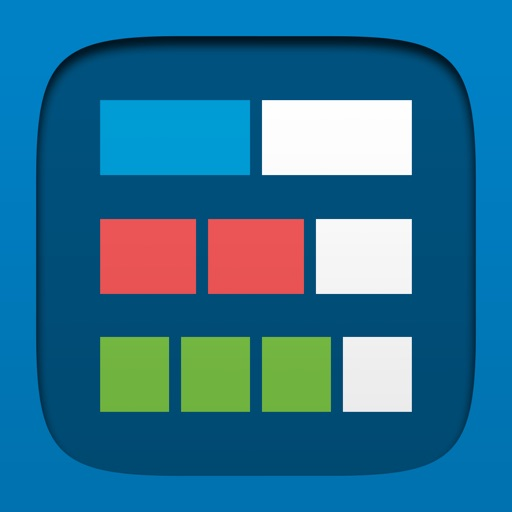## Fractions lets students use a bar or circle to represent, compare, and perform operations with fractions with denominators from 1 to 100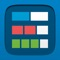# Fractions, by the Math Learning Center

by Clarity Innovations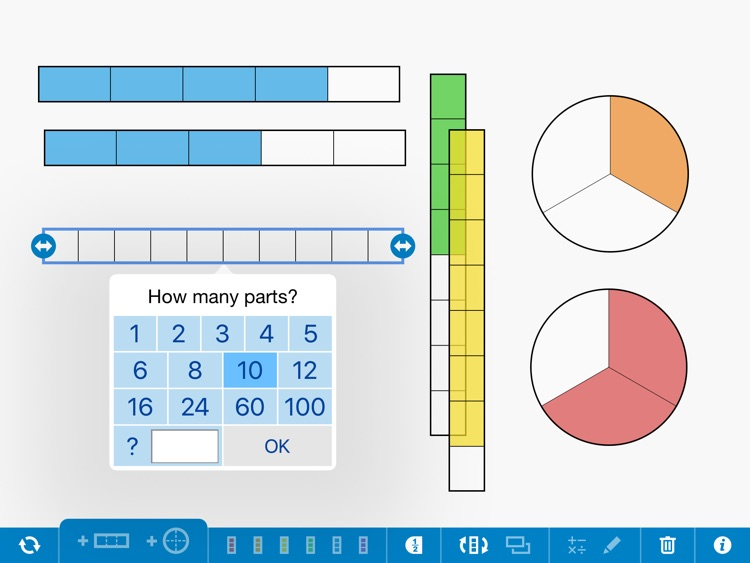Fractions lets students use a bar or circle to represent, compare, and perform operations with fractions with denominators from 1 to 100. Choose the fraction model and number of equal parts. Use a color to select specific parts to show a fraction of the whole. Reveal or hide numeric labels as needed. Superimpose fractions upon each other to compare fractions or see equal parts.Version
1.1.8
Rating
(5)
Size
2Mb
Genre
Education
Last updated
January 19, 2017
Release date
July 4, 2016

### App Screenshots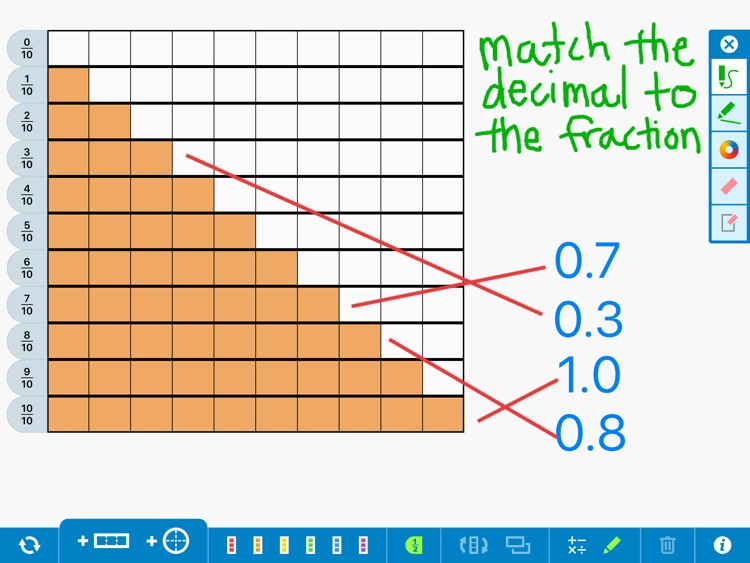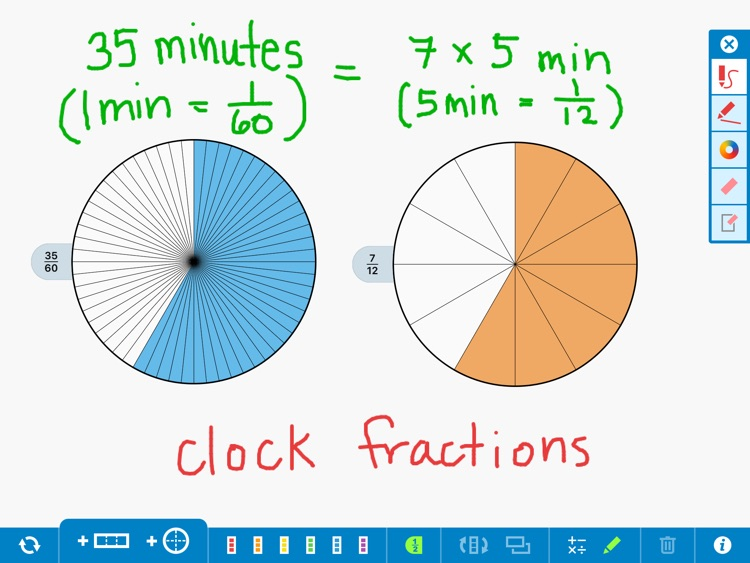### App Store Description

Fractions lets students use a bar or circle to represent, compare, and perform operations with fractions with denominators from 1 to 100. Choose the fraction model and number of equal parts. Use a color to select specific parts to show a fraction of the whole. Reveal or hide numeric labels as needed. Superimpose fractions upon each other to compare fractions or see equal parts.

FEATURES

• Use a bar or circle as the whole.
• Divide each whole into anywhere from 1 to 100 equal parts.
• Compare fractions and represent equivalent fractions.
• Add, subtract, multiply, and divide with fractions.
• Explore the relationship between fractions, percents, and decimals.
• Select the size of the whole and the number of equal parts.
• Hide and reveal fraction labels.
• Use the drawing tools to annotate work and show understanding.
• Write equations and expressions with the math text tool.